# NCERT Solutions for Class 8 Maths Chapter 6 Squares and Square Roots Ex 6.3

NCERT Solutions for Class 8 Maths Chapter 6 Squares and Square Roots Ex 6.3

### NCERT Solutions for Class 8 Maths Chapter 6 Squares and Square Roots Exercise 6.3

Ex 6.3 Class 8 Maths Question 1.
What could be the possible ‘one’s’ digits of the square root of each of the following numbers?
(i) 9801
(ii) 99856
(iii) 998001
(iv) 657666025
Solution:
(i) One’s digit in the square root of 9801 maybe 1 or 9.
(ii) One’s digit in the square root of 99856 maybe 4 or 6.
(iii) One’s digit in the square root of 998001 maybe 1 or 9.
(iv) One’s digit in the square root of 657666025 can be 5.

Ex 6.3 Class 8 Maths Question 2.
Without doing any calculation, find the numbers which are surely not perfect squares.
(i) 153
(ii) 257
(iii) 408
(iv) 441
Solution:
We know that the numbers ending with 2, 3, 7 or 8 are not perfect squares.
(i) 153 is not a perfect square number. (ending with 3)
(ii) 257 is not a perfect square number. (ending with 7)
(iii) 408 is not a perfect square number. (ending with 8)
(iv) 441 is a perfect square number.

Ex 6.3 Class 8 Maths Question 3.
Find the square roots of 100 and 169 by the method of repeated subtraction.
Solution:
Using the method of repeated subtraction of consecutive odd numbers, we have
(i) 100 – 1 = 99, 99 – 3 = 96, 96 – 5 = 91, 91 – 7 = 84, 84 – 9 = 75, 75 – 11 = 64, 64 – 13 = 51, 51 – 15 = 36, 36 – 17 = 19, 19 – 19 = 0
(Ten times repetition)
Thus √100 = 10

(ii) 169 – 1 = 168, 168 – 3 = 165, 165 – 5 = 160, 160 – 7 = 153, 153 – 9 = 144, 144 – 11 = 133, 133 – 13 = 120, 120 – 15 = 105, 105 – 17 = 88, 88 – 19 = 69, 69 – 21 = 48, 48 – 23 = 25, 25 – 25 = 0
(Thirteen times repetition)
Thus √169 = 13

Ex 6.3 Class 8 Maths Question 4.
Find the square roots of the following numbers by the prime factorisation Method.
(i) 729
(ii) 400
(iii) 1764
(iv) 4096
(v) 7744
(vi) 9604
(vii) 5929
(viii) 9216
(ix) 529
(x) 8100
Solution:
(i) We have 729
Prime factors of 729
729 = 3 × 3 × 3 × 3 × 3 × 3 = 32 × 32 × 32
√729 = 3 × 3 × 3 = 27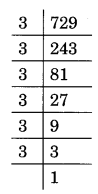(ii) We have 400
Prime factors of 400
400 = 2 × 2 × 2 × 2 × 5 × 5 = 22 × 22 × 52
√400 = 2 × 2 × 5 = 20(iii) 1764
1764 = 2 × 2 × 3 × 3 × 7 × 7 = 22 × 32 × 72
√1764 = 2 × 3 × 7 = 42(iv) 4096
4096 = 2 × 2 × 2 × 2 × 2 × 2 × 2 × 2 × 2 × 2 × 2 × 2
= 22 × 22 × 22 × 22 × 22 × 22
√4096 = 2 × 2 × 2 × 2 × 2 × 2 = 64(v) Prime factorisation of 7744 is
7744 = 2 × 2 × 2 × 2 × 2 × 2 × 11 × 11
= 22 × 22 × 22 × 112
√7744 = 2 × 2 × 2 × 11 = 88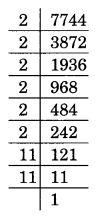(vi) Prime factorisation of 9604 is
9604 = 2 × 2 × 7 × 7 × 7 × 7 = 22 × 72 × 72
√9604 = 2 × 7 × 7 = 98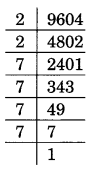(vii) Prime factorisation of 5929 is
5929 = 7 × 7 × 11 × 11 = 72 × 112
√5929 = 7 × 11 = 77(viii) Prime factorisation of 9216 is
9216 = 2 × 2 × 2 × 2 × 2 × 2 ×2 × 2 × 2 × 2 × 3 × 3
= 22 × 22 × 22 × 22 × 22 × 32
√9216 = 2 × 2 × 2 × 2 × 2 × 3 = 96(ix) Prime factorisation of 529 is
529 = 23 × 23 = 232
√529 = 23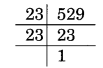(x) Prime factorisation of 8100 is
8100 = 2 × 2 × 3 × 3 × 3 × 3 × 5 × 5 = 22 × 32 × 32 × 52
√8100 = 2 × 3 × 3 × 5 = 90Ex 6.3 Class 8 Maths Question 5.
For each of the following numbers, find the smallest whole number by which it should be multiplied so as to get a perfect square number. Also, find the square root of the square number so obtained.
(i) 252
(ii) 180
(iii) 1008
(iv) 2028
(v) 1458
(vi) 768
Solution:
(i) Prime factorisation of 252 is
252 = 2 × 2 × 3 × 3 × 7
Here, the prime factorisation is not in pair. 7 has no pair.
Thus, 7 is the smallest whole number by which the given number is multiplied to get a perfect square number.
The new square number is 252 × 7 = 1764
Square root of 1764 is
√1764 = 2 × 3 × 7 = 42(ii) Primp factorisation of 180 is
180 = 2 × 2 × 3 × 3 × 5
Here, 5 has no pair.
New square number = 180 × 5 = 900
The square root of 900 is
√900 = 2 × 3 × 5 = 30
Thus, 5 is the smallest whole number by which the given number is multiplied to get a square number.(iii) Prime factorisation of 1008 is
1008 = 2 × 2 × 2 × 2 × 3 × 3 × 7
Here, 7 has no pair.
New square number = 1008 × 7 = 7056
Thus, 7 is the required number.
Square root of 7056 is
√7056 = 2 × 2 × 3 × 7 = 84(iv) Prime factorisation of 2028 is
2028 = 2 × 2 × 3 × 13 × 13
Here, 3 is not in pair.
Thus, 3 is the required smallest whole number.
New square number = 2028 × 3 = 6084
Square root of 6084 is
√6084 = 2 × 13 × 3 = 78(v) Prime factorisation of 1458 is
1458 = 2 × 3 × 3 × 3 × 3 × 3 × 3
Here, 2 is not in pair.
Thus, 2 is the required smallest whole number.
New square number = 1458 × 2 = 2916
Square root of 1458 is
√2916 = 3 × 3 × 3 × 2 = 54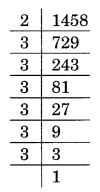(vi) Prime factorisation of 768 is
768 = 2 × 2 × 2 × 2 × 2 × 2 × 2 × 2 × 3
Here, 3 is not in pair.
Thus, 3 is the required whole number.
New square number = 768 × 3 = 2304
Square root of 2304 is
√2304 = 2 × 2 × 2 × 2 × 3 = 48Ex 6.3 Class 8 Maths Question 6.
For each of the following numbers, find the smallest whole number by which it should be divided so as to get a perfect square. Also, find the square root of the square number so obtained.
(i) 252
(ii) 2925
(iii) 396
(iv) 2645
(v) 2800
(vi) 1620
Solution:
(i) Prime factorisation of 252 is
252 = 2 × 2 × 3 × 3 × 7
Here 7 has no pair.
7 is the smallest whole number by which 252 is divided to get a square number.
New square number = 252 ÷ 7 = 36
Thus, √36 = 6(ii) Prime factorisation of 2925 is
2925 = 3 × 3 × 5 × 5 × 13
Here, 13 has no pair.
13 is the smallest whole number by which 2925 is divided to get a square number.
New square number = 2925 ÷ 13 = 225
Thus √225 = 15(iii) Prime factorisation of 396 is
396 = 2 × 2 × 3 × 3 × 11
Here 11 is not in pair.
11 is the required smallest whole number by which 396 is divided to get a square number.
New square number = 396 ÷ 11 = 36
Thus √36 = 6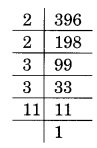(iv) Prime factorisation of 2645 is
2645 = 5 × 23 × 23
Here, 5 is not in pair.
5 is the required smallest whole number.
By which 2645 is multiplied to get a square number
New square number = 2645 ÷ 5 = 529
Thus, √529 = 23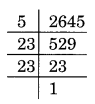(v) Prime factorisation of 2800 is
2800 = 2 × 2 × 2 × 2 × 5 × 5 × 7
Here, 7 is not in pair.
7 is the required smallest number.
By which 2800 is multiplied to get a square number.
New square number = 2800 ÷ 7 = 400
Thus √400 = 20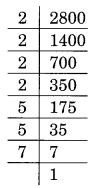(vi) Prime factorisation of 1620 is
1620 = 2 × 2 × 3 × 3 × 3 × 3 × 5
Here, 5 is not in pair.
5 is the required smallest prime number.
By which 1620 is multiplied to get a square number = 1620 ÷ 5 = 324
Thus √324 = 18Ex 6.3 Class 8 Maths Question 7.
The students of class VIII of a school donated ₹ 2401 in all, for Prime Minister’s National Relief Fund. Each student donated as many rupees as the number of students in the class. Find the number of students in the class.
Solution:
Total amount of money donated = ₹ 2401
Total number of students in the class = √2401Ex 6.3 Class 8 Maths Question 8.
2025 plants are to be planted in a garden in such a way that each row contains as many plants as the number of rows. Find the number of rows and the number of plants in each row.
Solution:
Total number of rows = Total number of plants in each row = √2025Thus the number of rows and plants = 45Ex 6.3 Class 8 Maths Question 9.
Find the smallest square number that is divisible by each of the numbers 4, 9 and 10.
Solution:
LCM of 4, 9, 10 = 180
The least number divisible by 4, 9 and 10 = 180
Now prime factorisation of 180 is
180 = 2 × 2 × 3 × 3 × 5
Here, 5 has no pair.
The required smallest square number = 180 × 5 = 900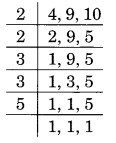Ex 6.3 Class 8 Maths Question 10.
Find the smallest number that is divisible by each of the numbers 8, 15 and 20.
Solution:
The smallest number divisible by 8, 15 and 20 is equal to their LCM.
LCM = 2 × 2 × 2 × 3 × 5 = 120
Here, 2, 3 and 5 have no pair.
The required smallest square number = 120 × 2 × 3 × 5 = 120 × 30 = 3600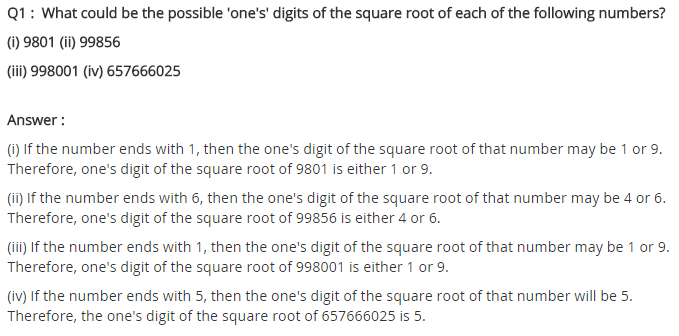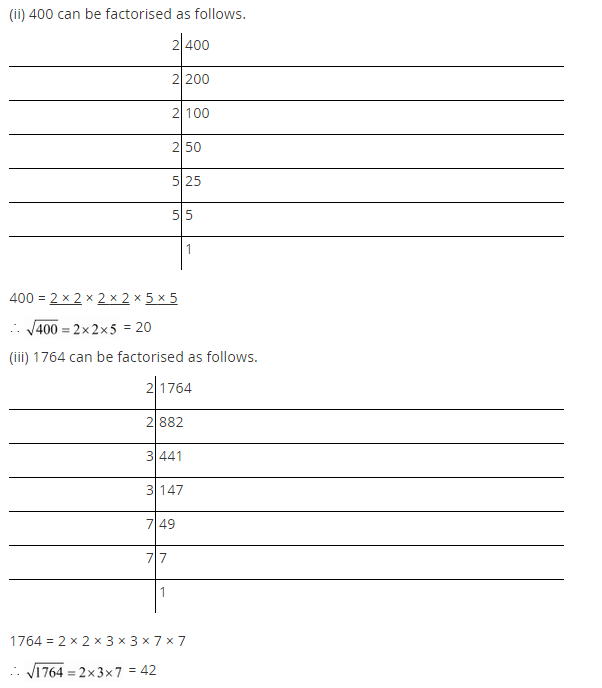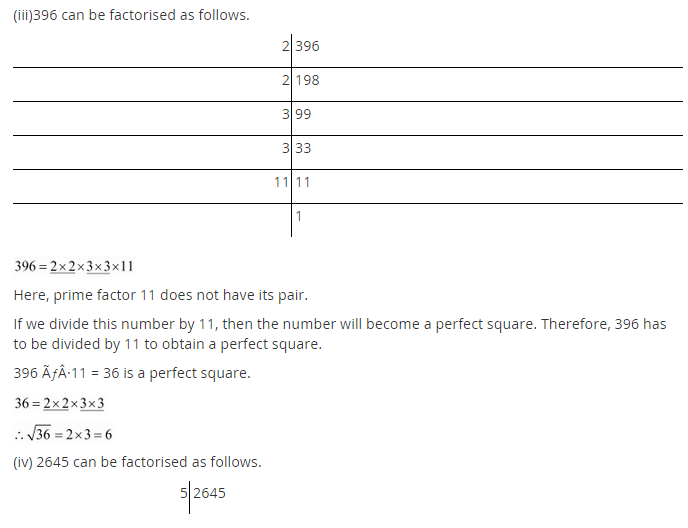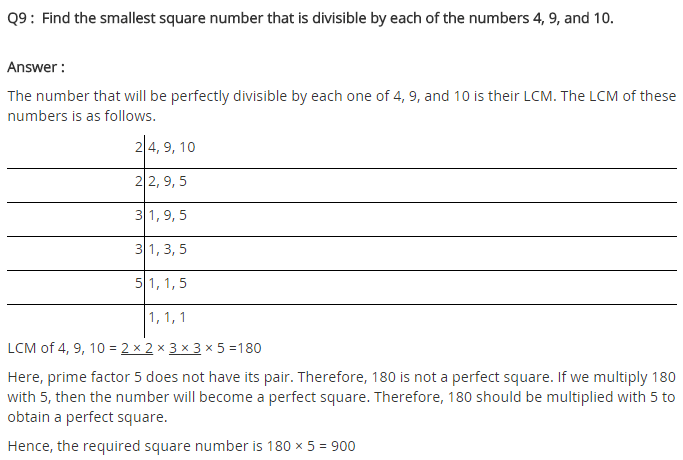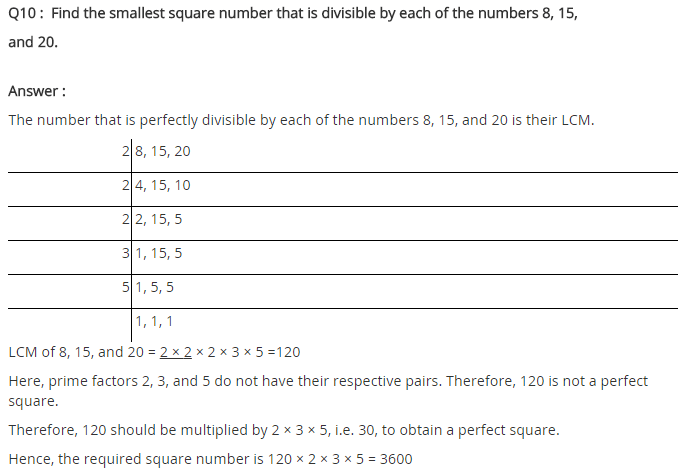## SabDekho

The Complete Educational Website